# Substring

There’s no SUBSTRING function in Excel. Use MID, LEFT, RIGHT, FIND, LEN, SUBSTITUTE, REPT, TRIM and MAX in Excel to extract substrings.

### Mid

To extract a substring, starting in the middle of a string, use the MID function in Excel.Explanation: the MID function starts at position 7 (O) and extracts 6 characters.

### Left

To extract the leftmost characters from a string, use the LEFT function in Excel.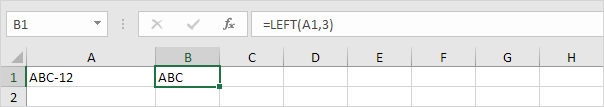To extract a substring (of any length) before the dash, add the FIND function.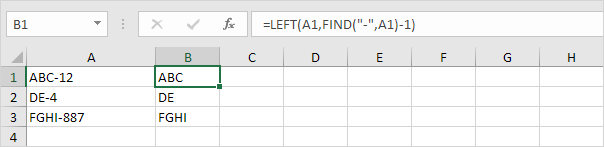Explanation: the FIND function finds the position of the dash. Subtract 1 from this result to extract the correct number of leftmost characters. The formula shown above reduces to LEFT(A1,4-1).

### Right

To extract the rightmost characters from a string, use the RIGHT function in Excel.To extract a substring (of any length) after the dash, add LEN and FIND.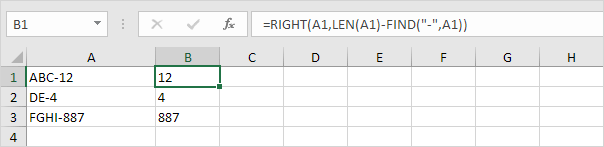Explanation: the LEN function returns the length of the string. The FIND function finds the position of the dash. Subtract these values to extract the correct number of rightmost characters. The formula shown above reduces to RIGHT(A1,6-4).

### Substring between parentheses

To extract a substring between parentheses (or braces, brackets, slashes, etc.), use MID and FIND in Excel.

1. The formula below is almost perfect.Explanation: the FIND function finds the position of the opening parenthesis. Add 1 to find the start position of the substring. The formula shown above reduces to MID(A1,6+1,2). This MID function always extracts 2 characters.

2. Replace the 2 (third argument) with a formula that returns the length of the substring.Explanation: subtract the position of the opening parenthesis and the value 1 from the position of the closing parenthesis to find the correct length of the substring.

### Substring containing specific text

To extract a substring containing specific text (for example, the @ symbol), use SUBSTITUTE, REPT, MID, FIND, TRIM and MAX in Excel.

1. First, use SUBSTITUTE and REPT to substitute a single space with 100 spaces (or any other large number).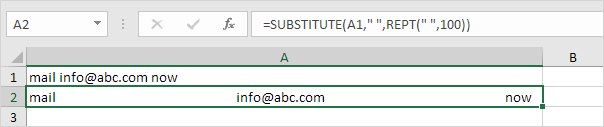2. To MID function below starts 50 (1/2 * large number) positions before the position of the @ symbol and extracts 100 (large number) characters.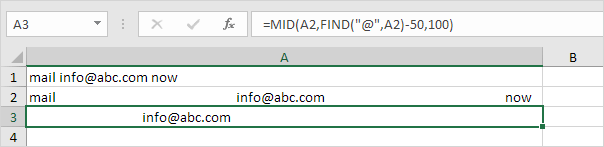3. Use the TRIM function to remove the leading and the trailing spaces.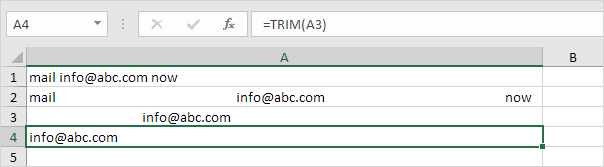4. Put it all together.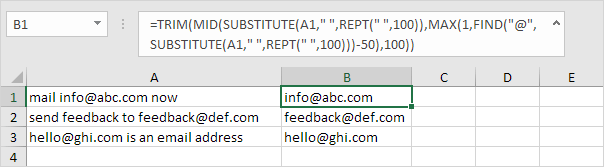Note: at step 2, the MID function starts 50 positions before the position of the @ symbol. If the email address is the first word in the sentence (cell A3), this results in a negative start position. In this case, the MAX function (see formula above) returns 1.

### Flash Fill

If you’re not a formula hero, use Flash Fill in Excel to automatically extract substrings.Note: Excel does not insert formulas, if you change the text strings in column A, Excel will not update the numbers in Column B.